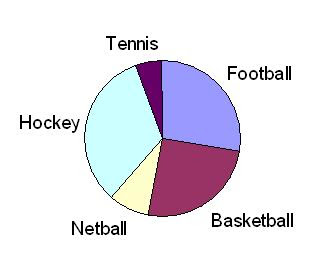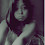## Sunday, April 19, 2009

### [Mathematic Form 3] Pie Chart

A pie chart can be used to represent statistical data. It is easy to remember the pie chart because, just like the most pies, it is in the shape of circle.

A pie chart is divided into sectors, whose angles at the center of circle are proportional to the frequency of a certain category of the data. The angles can also be represented as percentages in a pie chart.

To interpret the data in a pie chart, you have to:

i) Determine the angles at the center of each sector. The angle of each sector can be calculated by using the following formula:

Angle of sector = (Quantity represented by sector X 360o ) / Total quantity

ii) Note that the bigger an angle, the larger is the frequency or quantity represented
To convert the angle of a sector into a percentage, use the following formula:

Percentage = ( Angle of sector X 100% ) / 360o

Eg:The pie chart on the right represent the number of students who play basketball, football, tennis, hockey and netball in the third form of secondary school.

Football = 100o
Tennis = 20o
Netball = 30o
Hockey = x o

a) Find the value of x.
Solution:
x = 360o - 90o - 100o - 20o - 30o = 120o [Sum of angles at the center = 360o]

b) If the number of students who play netball is 45, calculate the total number of students in the third form.
Solution:
30o (the number of students who play netball) represent 45 students.
1o would represent (45/30) students

Hence the total number of students (represented by 360o) will be
= (45/30) X 360o
= 540 students

c) How much percentage of the total number of students in the third form play basketball?
Solution:
The percentage of students who play basketball
= (90/360) X 100%
= 25%

1.math is easy n simple !

2.but m3 its hards for me 2 undrstd?? =.= its really2 hard for me..

3.In This way its easy to understand....!

## Nota Terkini

• - Sumber-sumber tenaga yang terdapat di dunia ini adalah boleh dikelaskan kepada dua kumpulan, iaitu: 1. Sumber tenaga yang boleh diperbaharui. 2. Sumb...
• - *Hukum-hukum Indeks – Hukum 1* *am x** an= am+n* dimana *a*, *m* dan *n* adalah nombor nyata. *Pembuktian Hukum 1* *Contoh 1* Cari nilai bagi yang ber...
• - Formula am sabun dapat diwakili dengan RCOONa untuk garam natrium atau RCOOK untuk garam kalium dengan R sebagai kumpulan alkil yang berantai panjang. Co...
• - *Kepupusan haiwan* (animal extinction) bermaksud *lenyapnya* atau *sudah tiada lagi* sesuatu haiwan itu di muka bumi ini. Kewujudan haiwan-haiwan tersebut...
• - Apabila suatu voltan, *V*, dikenakan merentasi katod dan anod suatu senapang elektron, tenaga elektrik yang dibekalkan kepada setiap elektron ialah *eV*, d...